# IBPS Clerk Mains Quantitative Aptitude Questions 2019 (Day-8) High Level New Pattern

Dear Aspirants, Quantitative Aptitude plays a crucial role in Banking and all other competitive exams. To enrich your preparation, here we have provided New Pattern Aptitude Questions for IBPS Clerk Mains. Candidates those who are going to appear in IBPS Clerk Mains can practice these questions daily and make your preparation effective.

[WpProQuiz 4701]

Directions (1 – 5): Read the following information carefully and answer the given questions.

A survey was conducted in a school to find out what the students likes in their canteen for snacks. Each student likes only one of the following snacks. Once the survey complete, the surveyor built the Venn diagram.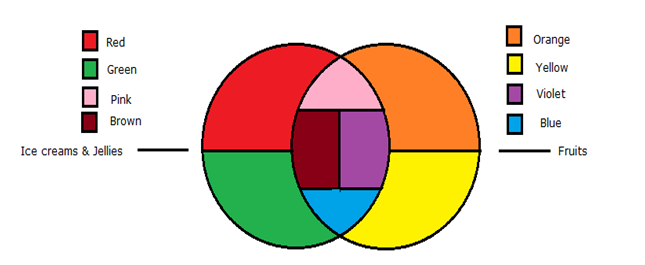The students like one out of exactly two types of fruits – apples (represented by the orange colour region in the fruits circle) and strawberries (represented by the yellow colour region in the fruits circle). They like one out of the ice creams and jellies – ice creams (represented by the red colour region in the circle) and jellies (represented by the green colour region in the circle). Some likes apple flavoured ice creams (represented by the pink colour region in the circle). Some likes strawberry flavoured ice creams (represented by violet colour region in the circle). Some likes apple flavoured jellies (represented by the brown colour region in the circle). Some likes strawberry flavoured jellies (represented by blue colour region in the circle). The following information also known,

1). 320 students likes only fruits and 240 students likes only ice cream (no flavoured).

2). 250 students like apples (either as fruit or flavoured ice cream or flavoured jellies).

3). 180 students like only jellies (no flavoured) and students like apple flavoured ice creams is 30 more than the apple flavoured jellies.

4). Students like strawberry flavoured jellies is half of the students like apple flavoured ice cream.

5). Students like strawberry flavoured ice cream is thrice the students like strawberry flavoured jellies.

6). Total students in the school is 1030.

1) Find the number of students like only strawberry?

a) 100

b) 120

c) 200

d) 300

e) 180

2) What is the ratio of the number of students like strawberry (either as fruits or flavoured ice cream or flavoured jellies) to the number of students like apple flavoured ice creams?

a) 4: 5

b) 9: 2

c) 3: 5

d) 7: 5

e) 2: 3

3) Number of students like Jellies is what percentage of the number of students like strawberry?

a) 60%

b) 50%

c) 80%

d) 75%

e) 90%

4) If the ratio of boys to girls like strawberry flavoured ice cream is 7: 5, then find the number of girls like strawberry flavoured ice cream

a) 40

b) 45

c) 80

d) 60

e) None of these

5) What is the difference between the number of students like flavoured ice cream and the flavoured jellies?

a) 110

b) 120

c) 130

d) 90

e) 80

Directions (6-8): Study the following graph carefully and answer the given questions.

Following table shows the total number of population in different villages and the percentage of female among them and the ratio of literate to illiterate population among them.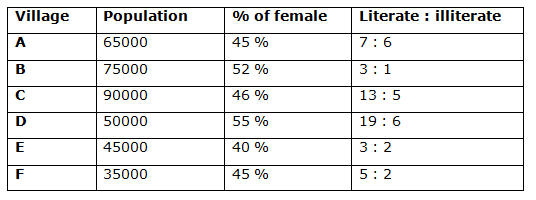6)

Quantity I: Find the total number of males in village B and C together?

Quantity II: Find the total number of literate people in village E and F together?

a) Quantity I > Quantity II

b) Quantity II > Quantity I

c) Quantity I ≥ Quantity II

d) Quantity II ≥ Quantity I

e) Quantity I = Quantity II (or) relationship cannot be determined

7)

Quantity I: Total illiterate people in village B and D together is approximately what percentage of the total illiterate people in village E and F together?

Quantity II: Total population in village F is what percentage of the total population in village A?

a) Quantity I > Quantity II

b) Quantity II > Quantity I

c) Quantity I ≥ Quantity II

d) Quantity II ≥ Quantity I

e) Quantity I = Quantity II (or) relationship cannot be determined

8)

Quantity I: Find the difference between the total females in all the given villages together and the total literate people in all the given villages together?

Quantity II: Find the difference between the total males in all the given villages together and the total illiterate people in all the given villages together?

a) Quantity I > Quantity II

b) Quantity II > Quantity I

c) Quantity I ≥ Quantity II

d) Quantity II ≥ Quantity I

e) Quantity I = Quantity II (or) relationship cannot be determined

Directions (9 -10): Study the following graph carefully and answer the given questions.

Train P started from station A towards station D with the speed of 40 Km/h at the same time train Q started from station D towards station A with the speed of 60 Km/h. All the four stations are in a straight line from left to right in the order A, B, C and D and distance between the consecutive stations is same (i.e.) 480 Km.

9) Which of the following statement is not true if train P and Q started from station A and B respectively at the end of 3 hours?

a) Train Q covered 60 km more than the train P

b) Train Q is 360 km far away from station C

c) Train P is 360 km far away from station B

d) The distance between train P and train Q is 1140 km

e) All are true

10) At what time train P and Q will meet each other?

a) 12 hours 20 minutes

b) 13 hours 30 minutes

c) 14 hours 20 minutes

d) 14 hours 24 minutes

e) None of these

Directions (1-5):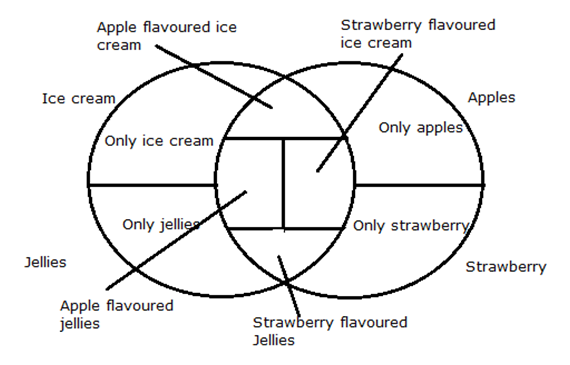Total students = 1030

From statement (3),

Students like Apple flavoured ice cream = 30 + Students like Apple flavoured jellies

Let us take students like apple flavoured jellies be x

Students like apple flavoured ice cream = x + 30

From statement (2),

Students like only apples = students like apples – students like apple flavoured ice cream – students like apple flavoured jellies

Students like only apples = 250 – x – (x+30)

= 250 – 2x – 30

= 220 – 2x

From statement (4),

Students like strawberry flavoured jellies = (½)*Students like apple flavoured ice cream

Students like strawberry flavoured jellies = ½*(x+30)

From statement (5),

Students like strawberry flavoured ice cream = 3 * students like strawberry flavoured jellies

= 3 * [(x+30)/2]

From statement (1),

Students likes only fruits = 320

Students likes only ice cream = 240

Students like only strawberry = 320 – (220 – 2x)

All the statements together we get,

(240+180+x+x+30+3/2(x+30) + (x+30)/2+220-2x+100+2x)= 1030

(770 + 2x+4/2(x+30)) = 1030

= > 2x + 2 (x+30) = 260

= > 2x + 2x +60 = 260

= > 4x = 260 – 60

= > 4x = 200

= > x = 50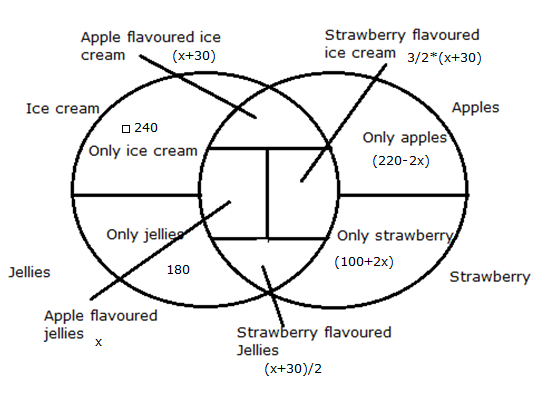Number of students like only strawberry = 200

Required ratio = (200+120+40): 80

= 360: 80

= 9: 2

Number of students like Jellies = (180+50+40)

= 270

Number of students like strawberry = (200+120+40)

= 360

Required percentage = 270/360 * 100

= ¾*100

= 75%

Number of students like strawberry flavoured ice cream = 120

Number of girls like strawberry flavoured ice cream = 120/12 * 5 = 50

Number of students like flavoured ice cream = (80+120)

= 200

Number of students like flavoured jellies = 50+40

= 90

Required difference = 200 – 90

= 110

Direction (6-8) :

Quantity I: Find the total number of males in village B and C together

Total males in village B and C together

= 75000 *(48/100) + 90000 *(54/100)

= 36000 + 48600

= 84600

Quantity II: Find the total number of literate people in village E and F together

Total number of literate people in village E and F together

= 45000 *(3/5) + 35000 *(5/7)

= 27000 + 25000

= 52000

Quantity I > Quantity II

Quantity I: Total illiterate people in village B and D together is approximately what percentage of the total illiterate people in village E and F together?

Total illiterate people in village B and D together

= 75000 *(1/4) + 50000 *(6/25)

= 18750 + 12000

= 30750

Total illiterate people in village E and F together

= 45000 *(2/5) + 35000 *(2/7)

= 18000 + 10000

= 28000

Required percentage = 30750/28000 *100

= 110%

Quantity II: Total population in village F is approximately what percentage of the total population in village A?

Required percentage = 35000/65000 * 100

= 54%

Quantity I > Quantity II

Quantity I: Find the difference between the total females in all the given villages together and the total literate people in all the given villages together

Total females in all the given villages together

= 65000 *(45/100) + 75000 *(52/100) + 90000 *(46/100) + 50000 *(55/100) + 45000 *(40/100) + 35000 *(45/100)

= 29250 + 39000 + 41400 + 27500 + 18000 + 15750

= 170900

Total literate people in all the given villages together

= 65000 *(7/13) + 75000 *(3/4) + 90000 *(13/18) + 50000 *(19/25) + 45000 *3/5) + 35000 *(5/7)

= 35000 + 56250 + 65000 + 38000 + 27000 + 25000

= 246250

Required difference = 246250 – 170900 = 75350

Quantity II: Find the difference between the total males in all the given villages together and the total illiterate people in all the given villages together

Total males in all the given villages together

= (65000+75000+90000+50000+45000+35000) – 170900

= 360000 – 170900

= 189100

Total illiterate people in all the given villages together

= (65000+75000+90000+50000+45000+35000) – 246250

= 360000 – 246250

= 113750

Required difference = 189100 – 113750 = 75350

Quantity I = Quantity II

Direction (9-10) :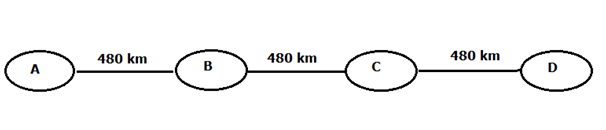Train P covered (3*40) = 120 km at the end of 3 hours

Train Q covered (3*60) = 180 km at the end of 3 hoursTotal distance = (480*3)/(40+60)

= 1440/100

= 14.4 hours (or) 14 hours 24 minutes